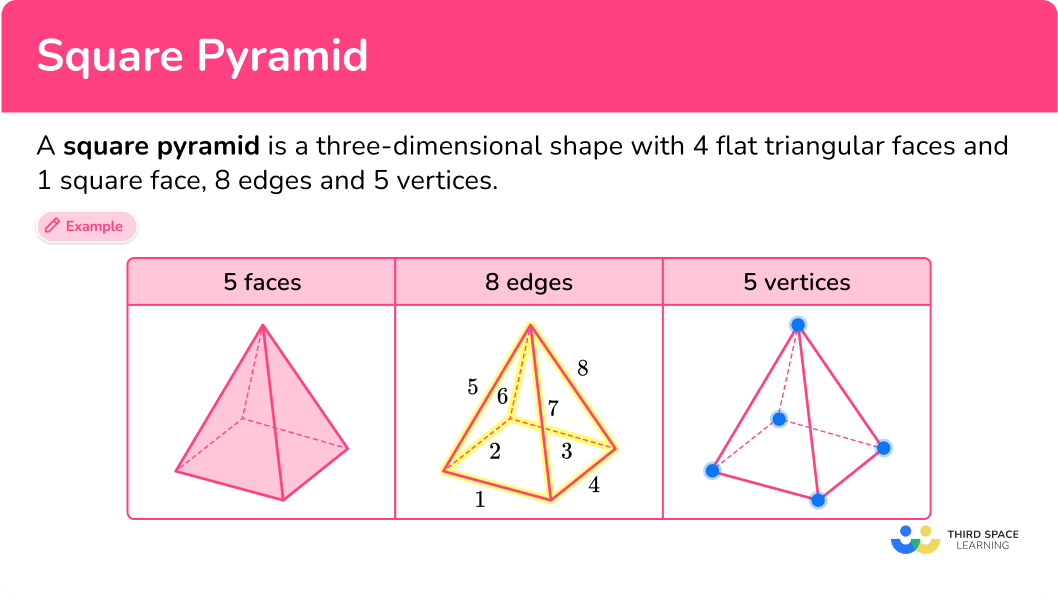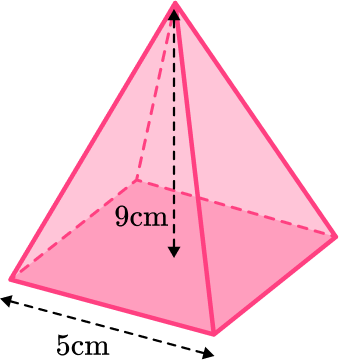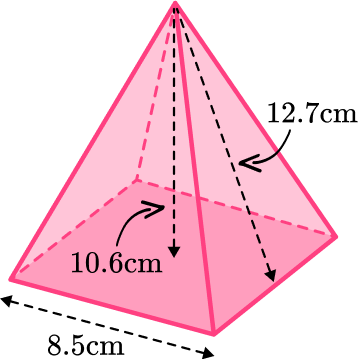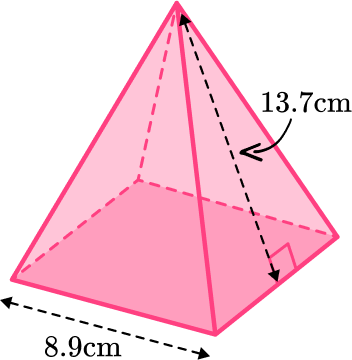Math resources Geometry

3D shapes

Square pyramid

# Square pyramid

Here you will learn about square pyramids, including what a square pyramid is and how to find the volume and surface area of square pyramids.

Students will first learn about square pyramids as part of geometry in 1 st grade. In middle school and high school, students will expand their knowledge of square pyramids when they learn to find the volume and the surface area of a square pyramid.

## What is a square pyramid?

A square pyramid is a three-dimensional shape with 4 flat triangular faces and 1 square face, 8 edges and 5 vertices. Rectangular based pyramids and triangular prisms are sometimes called pentahedrons because they have 5 faces.

\textbf{5} faces \textbf{8} edges \textbf{5} vertices

A pyramid is a polyhedra with a flat base and triangular sides which meet at the apex. The flat base can be any polygon. There are different types of pyramids such as a triangular pyramid (known as a tetrahedron), a rectangular pyramid or even a hexagonal pyramid or a pentagonal pyramid.

### Net of a square pyramid

The net of a square pyramid is made of 4 triangles and a square.

### Volume of a square pyramid

The volume of a square pyramid can be found by using the formula:

\text{Volume}=\cfrac{1}{3}\times \text{area of base} \times \text{height}

The pyramid height should be perpendicular to its base.

This can be written as,

V=\cfrac{1}{3} \, Bh

Where,

• V represents the volume of the pyramid.
• B represents the area of the base of the pyramid.
• h represents the perpendicular height of the pyramid.

### Surface area of a square pyramid

The surface area of a square pyramid is calculated by finding the area of each of the faces and adding them together.

For example, find the surface area of the square pyramid.

\text {Area of a square }=5 \mathrm{~cm} \times 5 \mathrm{~cm}=25 \mathrm{~cm}^2

\text {Area of a triangle }=\cfrac{5 \mathrm{~cm} \times 8 \mathrm{~cm}}{2}=20 \mathrm{~cm}^2

There are 4 triangular faces, so multiply 20 \mathrm{~cm}^2 by 4 to give 80 \mathrm{~cm}^2.

\text {Total surface area }=25 \mathrm{~cm}^2+80 \mathrm{~cm}^2=105 \mathrm{~cm}^2

### Types of square pyramids

There are two main types of square pyramids you will encounter, right square pyramids and oblique square pyramids.

A right square pyramid is a square pyramid where the apex is directly above the center of the square base, forming a right-angled triangle. The other 4 faces are congruent isosceles triangles.

An oblique square pyramid is a square pyramid where the apex is NOT directly above the center of the square base. The other 4 faces are triangles, but they are not all congruent.

### What is a square pyramid?## Common Core State Standards

How does this relate to 1 st grade and 7 th grade math?

Compose two-dimensional shapes (rectangles, squares, trapezoids, triangles, half-circles, and quarter-circles) or three-dimensional shapes (cubes, right rectangular prisms, right circular cones, and right circular cylinders) to create a composite shape, and compose new shapes from the composite shape.

Solve real-world and mathematical problems involving area, volume and surface area of two- and three-dimensional objects composed of triangles, quadrilaterals, polygons, cubes, and right prisms.

## How to identify a square pyramid

In order to identify a square pyramid, you will:

1. Look for the characteristics of a square pyramid.
2. State whether or not the shape is a square pyramid.
3. If the shape is not a square pyramid, explain what characteristics are different.

## Square pyramid examples

### Example 1: identifying square pyramid

Look at the image below and determine if it’s a square pyramid or not.

1. Look for the characteristics of a square pyramid.

A square pyramid is a 3D shape with 4 triangular faces and 1 square face, 8 edges and 5 vertices.

This shape has 5 triangular faces and 1 pentagonal face, 10 edges and 6 vertices.

2State whether or not the shape is a square pyramid.

This shape is not a square pyramid, it is a pentagonal pyramid.

3If the shape is not a square pyramid, explain what characteristics are different.

The pentagonal pyramid has a different number of faces ( 5 triangular faces and 1 pentagonal face), edges ( 10 edges) and vertices ( 6 vertices).

### Example 2: identifying square pyramid

Look at the image below and determine if it’s a square pyramid or not.

A square pyramid is a 3D shape with 4 triangular faces and 1 square face, 8 edges and 5 vertices.

This shape has 4 triangular faces and 1 square face, 8 edges and 5 vertices.

This shape is an oblique square pyramid.

## How to calculate the volume of a square pyramid

In order to calculate the volume of a square pyramid:

1. Calculate the area of the base.
2. Substitute values into the formula and solve.
3. Write the answer, including the units.

### Example 3: calculating the volume given height h

Find the volume of the square pyramid. Round the answer to the nearest tenth place.

The area of the base can be found by squaring the base side.

7^2=49

The volume of a square pyramid can be found by using the formula,

\begin{aligned} V&=\cfrac{1}{3} \, Bh \\\\ V&=\cfrac{1}{3}\times 49\times 10 \\\\ V&=163.333…\ \end{aligned}

The volume is 163.3{~m}^3, rounded to the tenth place.

### Example 4: calculating the height h given the volume

The volume of the square pyramid is 740 \mathrm{~cm}^3. Find the perpendicular height, h. Round the answer to two decimal places.

The area of the base can be found by multiplying the base edge by itself.

15 \times 15=225 \mathrm{~cm}^2

The formula for the volume of the square pyramid can be used to find the perpendicular height h.

\begin{aligned} V&=\cfrac{1}{3} \, Bh \\\\ 740&=\cfrac{1}{3}\times 225\times h \\\\ h&=\cfrac{740\times 3}{225}\\\\ h&=9.8666… \end{aligned}

The perpendicular height h is 9.87 \mathrm{~cm}, rounded to two decimal places.

## How to calculate the surface area of a square pyramid

In order to find the surface area of a square pyramid:

1. Calculate the area of each face.
2. Add the area of each face together.
3. Include the units.

### Example 5: working out the total surface area of a square pyramid

Find the total surface area of this square pyramid, where the apex is perpendicular to the center of the base.

The area of the base can be found by multiplying the base edge by itself.

8 \times 8=64 \mathrm{~cm}^2

The area of the triangular faces needs to be found. This is the lateral surface area.

You can find the area of one triangular face and then multiply it by four. You have the slant height which can be used to find the area of one triangular face. The triangular faces are all congruent isosceles triangles.

\begin{aligned} A&=\cfrac{1}{2} \, bh \\\\ A&=\cfrac{1}{2}\times 8\times 10 \\\\ A&=40 \end{aligned}

We can then multiply the area of one triangular face by 4 and add the area of the square base to find the total surface area.

4\times 40+64=224

The total surface area is 224 \mathrm{~cm}^2.

### Example 6: total surface area of a square pyramid

Find the total surface area of this right square pyramid.

The area of the base can be found by multiplying the base edge by itself.

6 \times 6=36 \mathrm{~cm}^2

The area of the triangular faces needs to be found. This is the lateral surface area.

You can find the area of one triangular face and then multiply it by four. You have the slant height which can be used to find the area of one triangular face. The triangular faces are all congruent isosceles triangles.

\begin{aligned} & A=\cfrac{1}{2} \, bh \\\\ & A=\cfrac{1}{2} \times 6 \times 9 \\\\ & A=27 \mathrm{~cm}^2 \end{aligned}

We can then multiply the area of one triangular face by 4 and add the area of the square base to find the total surface area.

4 \times 27+36=144

The total surface area of the pyramid is 144 \mathrm{~cm}^2.

### Teaching tips for square pyramids

• Have students show or describe real-life examples of square pyramids. This could include buildings, monuments, or everyday objects that resemble square pyramids.

• Using worksheets for practice is useful, but consider assigning group activities or problem-solving tasks related to square pyramids. Encourage students to share ideas, solve puzzles, or create their own problems involving square pyramids.

### Easy mistakes to make

• Not using the perpendicular height
The height of the pyramid needs to be the perpendicular height. This is the height that is at a right angle to the base.
For example, the triangle shows the perpendicular height of the right square pyramid.

• Using the incorrect units for volume and area
Remember to use cubic units for volume such as \mathrm{cm}^3 or \mathrm{m}^3. Square units are for area, for example \mathrm{cm}^2 or \mathrm{m}^2.

• Not using the correct formula when finding the volume
There are many different volume formulas in math. Make sure that you use the correct one to work out the volume of a pyramid.
\text{Volume}=\cfrac{1}{3}\times \text{area of base} \times \text{height}
Or
V=\cfrac{1}{3} \, Bh.

### Practice square pyramid questions

1) How many faces does a square pyramid have?

4375A square pyramid has 5 faces. One is the square base and the other 4 are triangles which are the lateral faces.

2) Which of these nets is for a square pyramid?A square pyramid has 5 faces. One is a square, the base. In a right pyramid, the other faces are triangles which need to have the same slant height so that they meet together at the apex.

3) Find the volume of this square pyramid.405 \mathrm{~cm}^3135 \mathrm{~cm}^375 \mathrm{~cm}^3225 \mathrm{~cm}^3Work out the volume of a pyramid by using the formula,

V=\cfrac{1}{3} \, Bh.

The base area can be found by multiplying the side length of the base square by itself.

5 \times 5=25

Substitute the values that have been given into the formula and work out the volume where,

B=25 \mathrm{~cm}^2 and h=9 \mathrm{~cm}.

V=\cfrac{1}{3}\times 25 \times 9=75

So the volume of the square pyramid is 75 \mathrm{~cm}^3.

4) Find the volume of this square pyramid to the nearest whole centimeter.255 \mathrm{~cm}^3305 \mathrm{~cm}^3256 \mathrm{~cm}^3306 \mathrm{~cm}^3Work out the volume of a pyramid by using the formula,

V=\cfrac{1}{3} \, Bh.

The base area can be found by multiplying the side length of the base square by itself.

8.5 \times 8.5=72.25

Substitute the values that have been given into the formula and work out the volume using the perpendicular height.

B=72.25 \mathrm{~cm}^2 and h=10.6 \mathrm{~cm}

V=\cfrac{1}{3}\times 72.25 \times 10.6=255.28…

The volume of the square pyramid is 255 \mathrm{~cm}^3 to the nearest centimeter.

5) The volume of a square pyramid is 165 \mathrm{~cm}^3. The side length of the square is 9.2 \mathrm{~cm}. Find the perpendicular height of the square pyramid. Round your answer to two decimal places.

5.84 \mathrm{~cm}7.33 \mathrm{~cm}5.85 \mathrm{~cm}7.34 \mathrm{~cm}Use the formula for volume of a pyramid,

V=\cfrac{1}{3} \, Bh.

The base area can be found by squaring the side length of the base square.

9.2^2=84.64

Substitute the values that have been given into the formula.

165=\cfrac{1}{3}\times 84.64 \times h

You can reverse this to find the base area.

h=\cfrac{165\times 3}{84.64}=5.8482…

The perpendicular height is 5.85 \mathrm{~cm} when rounded to 2 decimal places.

6) Find the total surface area of the square pyramid. The apex is directly above the center of the square base. Give your answer correct to the nearest whole number.323 \mathrm{~cm}^2432 \mathrm{~cm}^2140 \mathrm{~cm}^2253 \mathrm{~cm}^2A triangular based pyramid has 5 faces. The square base and 4 triangular faces.

Since the apex is directly above the center of the square base, all 4 triangles are congruent.

Find the area of the square base,

8.9 \times 8.9=79.21

Find the area of the triangular face,

A=\cfrac{1}{2} \, bh=\cfrac{1}{2}\times 8.9\times 13.7=60.965.

Finally, solve for the total surface area of the square pyramid,

79.21+4\times 60.965=323.07.

The total surface area of the square pyramid is 323 \mathrm{~cm}^2, rounded to the nearest whole number.

## Square pyramid FAQs

What is a square pyramid?

A square pyramid is a three-dimensional geometric shape with a square base and four triangular faces that meet at a single point called the apex or vertex. It is one type of pyramid.

Are the Pyramids of Egypt real-life examples of pyramids?

Yes, the Egyptian pyramids are examples of real-life pyramids. The Great Pyramid of Giza and The Pyramid of Djoser are examples of a square pyramids.

How is the Pythagorean Theorem related to square pyramids?

The Pythagorean theorem is used in square pyramids to calculate various measurements. It relates the lengths of the sides in a right triangle. In a square pyramid, the slant height, base length, and height form a right triangle.

The Pythagorean theorem can be applied to find the slant height, base side length, or height if the other two measurements are known.

## Still stuck?

At Third Space Learning, we specialize in helping teachers and school leaders to provide personalized math support for more of their students through high-quality, online one-on-one math tutoring delivered by subject experts.

Each week, our tutors support thousands of students who are at risk of not meeting their grade-level expectations, and help accelerate their progress and boost their confidence.